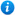# Comparing and Ordering Fractions - Year 5

KS2 Maths Teaching Resource: Comparing and Ordering Fractions - Year 5.

57 slide PowerPoint presentation and 12 worksheets.

Year 5 maths programme of study - Number - fractions (including decimals)

• compare and order fractions whose denominators are all multiples of the same number

'Comparing and Ordering Fractions - Year 5' is a KS2 maths teaching resource ideal to use in a lesson covering the year 5 curriculum objective listed above. This colourful and animated PowerPoint presentation can be used to introduce pupils to comparing and ordering fractions, or as a review of the topic. Content includes:

• A recap of what are fractions
• How to compare fractions with the same denominator
• How to compare fractions with the same numerator
• Comparing fractions with the same numerators and denominators activity and 2 differentiated worksheets
• How to compare fractions with different numerators and denominators
• Comparing fractions with different denominators activity and 2 differentiated worksheets.
• Comparing pairs of fractions activity
• How to order fractions with the same denominator
• Ordering fractions with the same denominator activity and worksheet
• How to order fractions with different denominators
• Ordering fractions by converting to an equivalent fraction with suggested common denominator activity and worksheet
• Ordering fractions with different denominators activity and worksheet
• Comparing fractions word problems and worksheet
• A link to a comparing fractions game
• 4 further differentiated worksheets

'Comparing and Ordering Fractions - Year 5' is fully editable so teachers are able to adapt the resource to suit the needs of each class they teach.

'Comparing and Ordering Fractions - Year 5' can be previewed in full by clicking on the PowerPoint images.

Our Price : £3.99 / 4 Credits## Get this resource as part of a bundle and save up to 70%## Year 5 Home Learning Pack 2

£15.99

By continuing to use the site, you agree to the use of cookies. You can change this and find out more by following this link